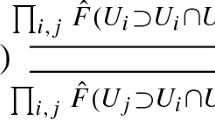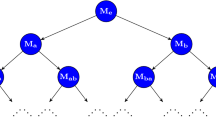# Generalized persistence diagrams

## Abstract

We generalize the persistence diagram of Cohen-Steiner, Edelsbrunner, and Harer to the setting of constructible persistence modules valued in a symmetric monoidal category. We call this the type $${\mathcal {A}}$$ persistence diagram of a persistence module. If the category is also abelian, then we define a second type $${\mathcal {B}}$$ persistence diagram. In addition, we show that both diagrams are stable to all sufficiently small perturbations of the module. The type $${\mathcal {B}}$$ persistence diagram carries less information than the type $${\mathcal {A}}$$ persistence diagram, but it enjoys a stronger stability theorem.

This is a preview of subscription content, log in via an institution to check access.

Price excludes VAT (USA)
Tax calculation will be finalised during checkout.

### Similar content being viewed by others### Homological Algebra for Persistence Modules

Peter Bubenik & Nikola Milićević### Persistence Diagrams as Diagrams: A Categorification of the Stability Theorem### The representation theorem of persistence revisited and generalized

René Corbet & Michael Kerber

1. The interval persistence module $${\mathsf {F}}_i$$ is fully described by the half open interval [st).

## References

• Anderson, F.W., Fuller, K.R.: Rings and Categories of Modules. Springer, New York (1992)

• Bubenik, P., de Silva, V., Nanda, V.: Higher interpolation and extension for persistence modules. SIAM J. Appl. Algebra Geom. 1, 272–284 (2017)

• Bubenik, P., de Silva, V., Scott, J.: Metrics for generalized persistence modules. Found. Comput. Math. 15(6), 1501–1531 (2015)

• Bender, EdA, Goldman, J.R.: On the applications of Möbius inversion in combinatorial analysis. Am. Math. Mon. 82(8), 789–803 (1975)

• Bubenik, P., Scott, J.: Categorification of persistent homology. Discrete Comput. Geom. 51(3), 600–627 (2014)

• Chazal, F., Cohen-Steiner, D., Glisse, M., Guibas, L., Oudot, S.: Proximity of persistence modules and their diagrams. In Proceedings of the 25th Annual Symposium on Computational Geometry, SCG ’09, pp. 237–246. ACM, New York (2009)

• Carlsson, G., de Silva, V.: Zigzag persistence. Found. Comput. Math. 10(4), 367–405 (2010)

• Chazal, F., de Silva, V., Glisse, M., Oudot, S.: The structure and stability of persistence modules. Springer International Publishing, Berlin (2016). https://www.springer.com/gb/book/9783319425436

• Cohen-Steiner, D., Edelsbrunner, H., Harer, J.: Stability of persistence diagrams. Discrete Comput. Geom. 37(1), 103–120 (2007)

• Curry, J.: Sheaves, cosheaves and applications. PhD thesis, University of Pennsylvania (2014)

• De Silva, V., Munch, E., Patel, A.: Categorified Reeb graphs. Discrete Comput. Geom. 55(4), 854–906 (2016)

• Edelsbrunner, H., Letscher, D., Zomorodian, A.: Topological persistence and simplification. Discrete Comput. Geom. 28(4), 511–533 (2002)

• Edelsbrunner, H., Morozov, D., Patel, A.: The Stability of the Apparent Contour of an Orientable 2-Manifold, pp. 27–41. Springer, Berlin (2011)

• Leinster, T.: Notions of Möbius inversion. Bull. Belg. Math. Soc. Simon Stevin 19(5), 909–933 (2012)

• Lesnick, M.: The theory of the interleaving distance on multidimensional persistence modules. Found. Comput. Math. 15(3), 613–650 (2015)

• Morozov, D., Beketayev, K., Weber, G.: Interleaving distance between merge trees. In: Proceedings of TopoInVis 2013 (2013)

• Mitchell, B.: Theory of Categories. Academic Press, Boston (1965)

• Rota, G.C.: On the foundations of combinatorial theory I. Theory of Möbius functions. Zeitschrift für Wahrscheinlichkeitstheorie und Verwandte Gebiete 2(4), 340–368 (1964)

• Weibel, C.A.: An Introduction to Homological Algebra. Cambridge University Press, Cambridge (1995)

• Weibel, C.A.: The K-book: an introduction to algebraic K-theory. American Mathematical Society, Providence (2013)

• Zomorodian, A., Carlsson, G.: Computing persistent homology. Discrete Comput. Geom. 33(2), 249–274 (2005)

## Acknowledgements

We thank Robert MacPherson for his mentorship and support. We thank Vin de Silva for detailed comments on earlier versions of this paper. We also thank the participants of the MacPherson Seminar on applied topology for listening and providing helpful feedback. Finally, we thank our anonymous reviewers for their patience and transformative feedback. This material is based upon work supported by the National Science Foundation under agreement no. DMS-1128155. Any opinions, findings and conclusions or recommendations expressed in this material are those of the author and do not necessarily reflect the views of the National Science Foundation.

## Author information

Authors

### Corresponding author

Correspondence to Amit Patel.

## Appendix: Krull-Schmidt

### Appendix: Krull-Schmidt

We now provide a compact treatment of Krull-Schmidt categories. The following ideas are classical and may be found in many books, for example Anderson and Fuller (1992).

A category $${\mathsf {C}}$$ is additive if all its hom-sets are abelian, composition is bilinear, and finite products and finite coproducts are the same. The (co)product of the empty set is the zero object of $${\mathsf {C}}$$. Suppose $${\mathsf {C}}$$ is additive.

### Definition A.1

A non-zero object $$a \in {\mathsf {C}}$$ is indecomposable if it is not the direct sum of two non-zero objects.

### Definition A.2

An additive category $${\mathsf {C}}$$ is Krull-Schmidt if each object $$a \in {\mathsf {C}}$$ is isomorphic to a finite direct sum $$a \cong a_1 \oplus a_2 \oplus \cdots \oplus a_n$$ and each ring of endomorphisms $${\mathsf {End}}_{\mathsf {C}}(a_i)$$ is local. That is, $$0 \ne 1$$ and if $$f_1 + f_2 = 1$$, then $$f_1$$ or $$f_2$$ is invertible.

Suppose $${\mathsf {C}}$$ is Krull-Schmidt.

### Proposition 9.1

An object $$a \in {\mathsf {C}}$$ is indecomposable iff its endomorphism ring $${\mathsf {End}}(a)$$ is local.

### Proof

Suppose $$a \in {\mathsf {C}}$$ is decomposable. That is, there is an isomorphism $$i :a \rightarrow a_1 \oplus a_2$$ such that $$a_1,a_2 \ne 0$$. Define $$\pi _1 : a_1 \oplus a_2 \rightarrow a_1 \oplus a_2$$ as the endomorphism that sends the first factor to zero and $$\pi _2 : a_1 \oplus a_2 \rightarrow a_1 \oplus a_2$$ as the endomorphism that sends the second factor to zero. Then the two maps $$\rho _1, \rho _2 : a \rightarrow a$$, where $$\rho _1 = i^{-1} \circ \pi _1 \circ i$$ and $$\rho _2 = i^{-1} \circ \pi _2 \circ i$$, are both non-isomorphisms in $${\mathsf {End}}_{\mathsf {C}}(a)$$. However, $$\rho _0 + \rho _1 :a \rightarrow a$$ is an isomorphism. We have a contradiction of locality.

Suppose $$a \in {\mathsf {C}}$$ is indecomposable. Then, by definition of a Krull-Schmidt category, $${\mathsf {End}}_{\mathsf {C}}(a)$$ is a local ring. $$\square$$

### Proposition 9.2

Each object $$a \in {\mathsf {C}}$$ is isomorphic to a finite direct sum of indecomposables.

### Proof

By definition of a Krull-Schmidt category, $$a \cong a_1 \oplus a_2 \oplus \cdots \oplus a_n$$ where each $${\mathsf {End}}_{\mathsf {C}}(a_i)$$ is a local ring. By Proposition 9.1, each $$a_i$$ is indecomposable. $$\square$$

### Theorem 9.1

(Krull-Schmidt) Suppose an object $$c \in {\mathsf {C}}$$ is isomorphic to $$a_1 \oplus a_2 \oplus \cdots \oplus a_m$$ and $$b_1 \oplus b_2 \oplus \cdots \oplus b_n$$, where each $$a_i$$ and $$b_j$$ are indecomposable. Then $$m = n$$, and there is a permutation $$p: [m] \rightarrow [n]$$ such that $$a_i \cong b_{p(i)}$$.

### Proof

By definition of an additive category, we have canonical projections $$\pi _i : \oplus _i a_i \rightarrow a_i$$ and $$\rho _j : \oplus _j b_j \rightarrow b_j$$ and canonical inclusions $$\mu _i : a_i \rightarrow \oplus _i a_i$$ and $$\nu _j : b_j \rightarrow \oplus _j b_j$$. Furthermore $$\mu _j \circ \pi _i$$ and $$\nu _j \circ \rho _i$$ are the identity on $$a_i$$ and $$b_i$$, respectively, iff $$i = j$$. Let $$f : a_1 \oplus a_2 \oplus \cdots \oplus a_m \rightarrow b_1 \oplus b_2 \oplus \cdots \oplus b_n$$ be an isomorphism.

Define $$h_j : a_1 \rightarrow a_1$$ as $$h_j = \pi _1 \circ f^{-1} \circ \nu _j \circ \rho _j \circ f \circ \mu _1$$. Let $$h = \sum _j h_j: a_1 \rightarrow a_1$$. Observe h is an isomorphism. By locality, there is an index j such that $$h_j$$ is an isomorphism. This means $$a_1 \cong b_j$$ and we specify $$p(1) = j$$. Quotient by $$a_1$$ and $$b_j$$. Repeat. $$\square$$

## Rights and permissions

Reprints and Permissions